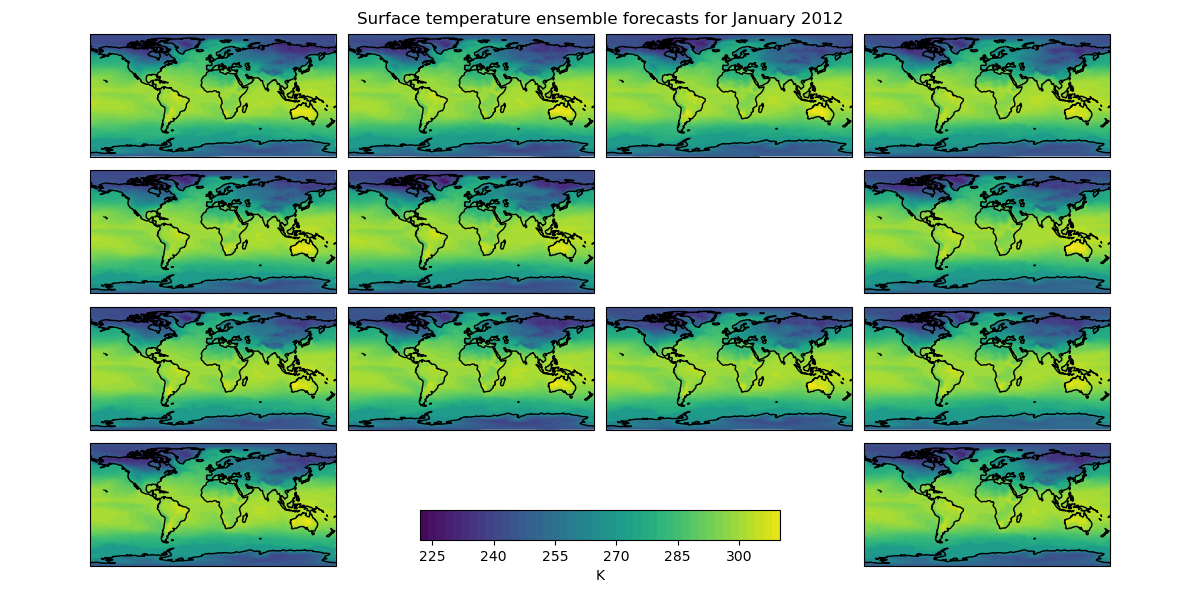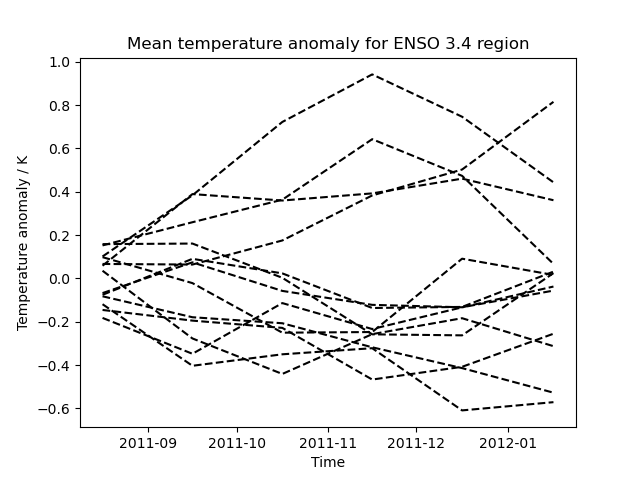# Seasonal Ensemble Model Plots#

This example demonstrates the loading of a lagged ensemble dataset from the GloSea4 model, which is then used to produce two types of plot:

• The first shows the “postage stamp” style image with an array of 14 images, one for each ensemble member with a shared colorbar. (The missing image in this example represents ensemble member number 6 which was a failed run)

• The second plot shows the data limited to a region of interest, in this case a region defined for forecasting ENSO (El Nino-Southern Oscillation), which, for the purposes of this example, has had the ensemble mean subtracted from each ensemble member to give an anomaly surface temperature. In practice a better approach would be to take the climatological mean, calibrated to the model, from each ensemble member.

••```import matplotlib.pyplot as plt
import matplotlib.ticker
import numpy as np

import iris
import iris.plot as iplt

"""
(ensemble member) coordinate from the filename if one doesn't already exist
in the cube.

"""
if not cube.coords("realization"):
# The ensemble member is encoded in the filename as *_???.pp where ???
# is the ensemble member.
realization_number = fname[-6:-3]
realization_coord = iris.coords.AuxCoord(
np.int32(realization_number), "realization", units="1"
)

def main():
# Create a constraint to extract surface temperature cubes which have a
# "realization" coordinate.
constraint = iris.Constraint(
"surface_temperature", realization=lambda value: True
)
# Use this to load our ensemble.  The callback ensures all our members
# have the "realization" coordinate and therefore they will all be loaded.
iris.sample_data_path("GloSea4", "ensemble_???.pp"),
constraint,
)

# -------------------------------------------------------------------------
# Plot #1: Ensemble postage stamps
# -------------------------------------------------------------------------

# For the purposes of this example, take the last time element of the cube.
# First get hold of the last time by slicing the coordinate.
last_time_coord = surface_temp.coord("time")[-1]
last_timestep = surface_temp.subset(last_time_coord)

# Find the maximum and minimum across the dataset.
data_min = np.min(last_timestep.data)
data_max = np.max(last_timestep.data)

# Create a wider than normal figure to support our many plots.
plt.figure(figsize=(12, 6), dpi=100)

# Also manually adjust the spacings which are used when creating subplots.
hspace=0.05,
wspace=0.05,
top=0.95,
bottom=0.05,
left=0.075,
right=0.925,
)

# Iterate over all possible latitude longitude slices.
for cube in last_timestep.slices(["latitude", "longitude"]):
# Get the ensemble member number from the ensemble coordinate.
ens_member = cube.coord("realization").points

# Plot the data in a 4x4 grid, with each plot's position in the grid
# being determined by ensemble member number.  The special case for the
# 13th ensemble member is to have the plot at the bottom right.
if ens_member == 13:
plt.subplot(4, 4, 16)
else:
plt.subplot(4, 4, ens_member + 1)

# Plot with 50 evenly spaced contour levels (49 intervals).
cf = iplt.contourf(cube, 49, vmin=data_min, vmax=data_max)

plt.gca().coastlines()

# Make an axes to put the shared colorbar in.
colorbar_axes = plt.gcf().add_axes([0.35, 0.1, 0.3, 0.05])
colorbar = plt.colorbar(cf, colorbar_axes, orientation="horizontal")
colorbar.set_label(last_timestep.units)

# Limit the colorbar to 8 tick marks.
colorbar.locator = matplotlib.ticker.MaxNLocator(8)
colorbar.update_ticks()

# Get the time for the entire plot.
time = last_time_coord.units.num2date(last_time_coord.bounds[0, 0])

# Set a global title for the postage stamps with the date formatted by
# "monthname year".
time_string = time.strftime("%B %Y")
plt.suptitle(f"Surface temperature ensemble forecasts for {time_string}")

iplt.show()

# -------------------------------------------------------------------------
# Plot #2: ENSO plumes
# -------------------------------------------------------------------------

# Nino 3.4 lies between: 170W and 120W, 5N and 5S, so use the intersection
# method to restrict to this region.
nino_cube = surface_temp.intersection(
latitude=[-5, 5], longitude=[-170, -120]
)

# Calculate the horizontal mean for the nino region.
mean = nino_cube.collapsed(["latitude", "longitude"], iris.analysis.MEAN)

# Calculate the ensemble mean of the horizontal mean.
ensemble_mean = mean.collapsed("realization", iris.analysis.MEAN)

# Take the ensemble mean from each ensemble member.
mean -= ensemble_mean

plt.figure()

for ensemble_member in mean.slices(["time"]):
# Draw each ensemble member as a dashed line in black.
iplt.plot(ensemble_member, "--k")

plt.title("Mean temperature anomaly for ENSO 3.4 region")
plt.xlabel("Time")
plt.ylabel("Temperature anomaly / K")

iplt.show()

if __name__ == "__main__":
main()
```

Total running time of the script: (0 minutes 30.443 seconds)

Gallery generated by Sphinx-Gallery